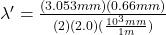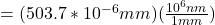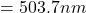## Light of wavelength 710 nm passes through two narrow slits 0.66 mm apart. The screen is 2.00 m away. A second source of unknown wavelength p

Question

Light of wavelength 710 nm passes through two narrow slits 0.66 mm apart. The screen is 2.00 m away. A second source of unknown wavelength produces its second-order fringe 1.25 mm closer to the central maximum than the 710-nm light. What is the wavelength of unknown light?

in progress 0
6 months 2021-09-04T19:58:12+00:00 1 Answers 0 views 0

The wavelength is 503 nm

Explanation:

Considering constructive interference , this means that route(path) difference is equal to the product of order of fringe and wavelength of the light

i.e   dsinθ  = m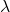Whereis the wavelength of light  and m is the order of the fringe

Looking at θ to be very small , sin θ can be approximated to  θ

and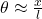Substituting this into the above equation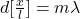making x the subject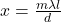This above equation will give the value of the distance of the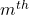order fringe of the wavelengthfrom the central fringe

Replacing with the value given in the question we have= 710 nm  m = 2  d =0.66 mm , l = 2.0 m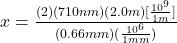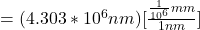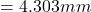The separation of the second fringe from central maximum is 4,303 mm

To obtain the separation of the second order fringe of the unknown light from central maximum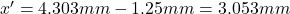Now to obtain the wavelength of this second source

from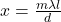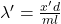Now substituting 3,053 mm for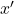2.0 mm for l , 0.66 mm  for d and 2 for m in the above formula